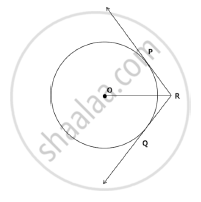# In the below given figure, two tangents RQ and RP are drawn from an external point R to the circle with centre O. - Mathematics

In the below given figure, two tangents RQ and RP are drawn from an external point R to the circle with centre O. If∠PRQ = 120°, then prove that OR = PR + RQ.#### Solution

Given that m∠PRQ = 120ºWe know that the line joining the centre and the external point is the angle bisector between the tangents.
Thus, m∠PRO = m∠QRO =120º/2= 60º
Also we know that lengths of tangents from an external point are equal.

Thus, PR = RQ.
Join OP and OQ.
Since OP and OQ are the radii from the centre O,
OP ⊥ PR and OQ ⊥ RQ.

Thus, Δ OPR and ΔOQR are right angled congruent triangles.

Hence, ∠POR=90º- ∠PRO=90º - 60º= 30º

∠QOR=90°- ∠QRO=90° - 60° =30°

sin ∠QRO = sin30°=1/2

Thus,PR/OR=1/ 2
OR =2PR
OR =PR + PR
OR =PR + QR

Concept: Number of Tangents from a Point on a Circle
Is there an error in this question or solution?